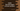# Kotlin program to find out the largest among three numbers# Introduction :

In this Kotlin programming tutorial, we will learn how to find out the largest number among the three. The user will enter the number values, our program will find and print out the result.

To compare three numbers and finding out the largest one, we can either implement our own algorithm or we can use Kotlin’s built-in method maxOf. In this tutorial, we will learn how to solve this problem with both approaches.

Find out the largest using when block :

We can either use if-else statement or when block to find out the largest among three numbers. Let’s check how to do this with a when block :

``````fun findLargest(first: Int, second: Int, third: Int): Int {
//4
return when {
first >= second && first >= third -> first
second >= first && second >= third -> second
else -> third
}
}

fun main(args: Array) {
//1
var firstNo: Int
var secondNo: Int
var thirdNo: Int

//2
print("Enter the first number : ")
firstNo = readLine()?.toInt() ?: -1

print("Enter the second number : ")
secondNo = readLine()?.toInt() ?: -1

print("Enter the third number : ")
thirdNo = readLine()?.toInt() ?: -1

//3
println("Largest among \$firstNo,\$secondNo and \$thirdNo is \${findLargest(firstNo, secondNo, thirdNo)}")
}``````

### Sample Output :

``````Enter the first number : 34
Enter the second number : 12
Enter the third number : 44
Largest among 34,12 and 44 is 44

Enter the first number : 456
Enter the second number : 100
Enter the third number : 1
Largest among 456,100 and 1 is 456``````

### Explanation :

The commented numbers in the above program denote the step numbers below :

1. Create three Int variables firstNo, secondNo and thirdNo.
2. Ask the user to enter the first number. Read it and store it in firstNo variable. Similarly, read the second and the third number and store in secondNo and thirdNo variables.
3. Find out the largest among these numbers using findLargest method. This method takes three Int arguments and returns one Int value, i.e. the largest among the arguments.
4. We are using one when block here to compare all three arguments and it is returning the largest one.

Find out the largest using maxOf :

Instead of using a when block, we can also use maxOf method to find out the largest. This method comes with the following variations for three arguments :

``````fun maxOf(a: Byte, b: Byte, c: Byte): Byte
fun maxOf(a: Short, b: Short, c: Short): Short
fun maxOf(a: Int, b: Int, c: Int): Int
fun maxOf(a: Long, b: Long, c: Long): Long
fun maxOf(a: Float, b: Float, c: Float): Float
fun maxOf(a: Double, b: Double, c: Double): Double``````

We can also use it with Comparable :

``````fun > maxOf(a: T, b: T, c: T): T
fun  maxOf(a: T, b: T, c: T, comparator: Comparator): T``````

As you can see, we can use this method for any data type that we want. For using this method, change the findLargest function as below :

``````fun findLargest(first: Int, second: Int, third: Int): Int {
return maxOf(first,second,third)
}``````

### Conclusion :

As you can see, for finding out the largest number in Kotlin, we can either write our own function or we can use the inbuilt maxOf. Try to run the above examples and drop one comment below if you have any queries.